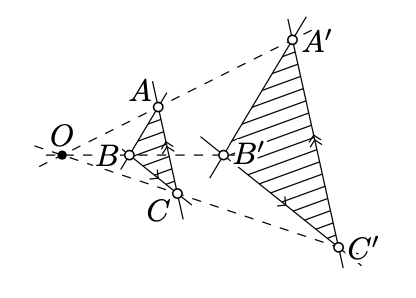Skip to main content
$$\newcommand{\id}{\mathrm{id}}$$ $$\newcommand{\Span}{\mathrm{span}}$$ $$\newcommand{\kernel}{\mathrm{null}\,}$$ $$\newcommand{\range}{\mathrm{range}\,}$$ $$\newcommand{\RealPart}{\mathrm{Re}}$$ $$\newcommand{\ImaginaryPart}{\mathrm{Im}}$$ $$\newcommand{\Argument}{\mathrm{Arg}}$$ $$\newcommand{\norm}{\| #1 \|}$$ $$\newcommand{\inner}{\langle #1, #2 \rangle}$$ $$\newcommand{\Span}{\mathrm{span}}$$

# 15.6: Moving points to infinity

$$\newcommand{\vecs}{\overset { \rightharpoonup} {\mathbf{#1}} }$$ $$\newcommand{\vecd}{\overset{-\!-\!\rightharpoonup}{\vphantom{a}\smash {#1}}}$$$$\newcommand{\id}{\mathrm{id}}$$ $$\newcommand{\Span}{\mathrm{span}}$$ $$\newcommand{\kernel}{\mathrm{null}\,}$$ $$\newcommand{\range}{\mathrm{range}\,}$$ $$\newcommand{\RealPart}{\mathrm{Re}}$$ $$\newcommand{\ImaginaryPart}{\mathrm{Im}}$$ $$\newcommand{\Argument}{\mathrm{Arg}}$$ $$\newcommand{\norm}{\| #1 \|}$$ $$\newcommand{\inner}{\langle #1, #2 \rangle}$$ $$\newcommand{\Span}{\mathrm{span}}$$ $$\newcommand{\id}{\mathrm{id}}$$ $$\newcommand{\Span}{\mathrm{span}}$$ $$\newcommand{\kernel}{\mathrm{null}\,}$$ $$\newcommand{\range}{\mathrm{range}\,}$$ $$\newcommand{\RealPart}{\mathrm{Re}}$$ $$\newcommand{\ImaginaryPart}{\mathrm{Im}}$$ $$\newcommand{\Argument}{\mathrm{Arg}}$$ $$\newcommand{\norm}{\| #1 \|}$$ $$\newcommand{\inner}{\langle #1, #2 \rangle}$$ $$\newcommand{\Span}{\mathrm{span}}$$

Theorem 15.5.1 makes it possible to take any line in the projective plane and declare it to be ideal. In other words, we can choose a preferred affine plane by removing one line from the projective plane. This construction provides a method for solving problems in projective geometry which will be illustrated by the following classical example:

Theorem $$\PageIndex{1}$$ Desargues' theorem

Desargues’ theorem[thm:desargues] Consider three concurrent lines $$(AA')$$, $$(BB')$$, and $$(CC')$$ in the real projective plane. Set

\begin{aligned} X&=(BC)\cap (B'C'),\\ Y&=(CA)\cap (C'A'),\\ Z&=(AB)\cap (A'B').\end{aligned}

Then the points $$X$$, $$Y$$, and $$Z$$ are collinear.Proof

Without loss of generality, we may assume that the line $$(XY)$$ is ideal. If not, apply a perspective projection that sends the line $$(XY)$$ to the ideal line.That is, we can assume that

$$(BC) \parallel (B'C')\ \ \ \ \text{and} \ \ \ \ (CA) \parallel (C'A')$$

and we need to show that

$$(AB) \parallel(A'B').$$

Assume that the lines $$(AA')$$, $$(BB')$$, and $$(CC')$$ intersect at point $$O$$. Since $$(BC) \parallel (B'C')$$, the transversal property (Theorem 7.3.1) implies that $$\measuredangle OBC = \measuredangle OB'C'$$ and $$\measuredangle OCB = \measuredangle OC'B'$$. By the AA similarity condition, $$\triangle OBC\z\sim\triangle OB'C'$$. In particular,

$$\dfrac{OB}{OB'}=\dfrac{OC}{OC'}.$$

The same way we get that $$\triangle OAC \sim\triangle OA'C'$$ and

$$\dfrac{OA}{OA'}=\dfrac{OC}{OC'}.$$

Therefore,

$$\dfrac{OA}{OA'}=\dfrac{OB}{OB'}.$$

By the SAS similarity condition, we get that $$\triangle OAB\sim\triangle OA'B'$$; in particular, $$\measuredangle OAB = \pm\measuredangle OA'B'$$.

Note that $$\measuredangle AOB=\measuredangle A'OB'$$. Therefore,

$$\measuredangle OAB=\measuredangle OA'B'.$$

By the transversal property (Theorem 7.3.1), we have $$(AB)\parallel (A'B')$$.

The case $$(AA') \parallel (BB') \parallel (CC')$$ is done similarly. In this case the quadrangles $$B'BCC'$$ and $$A'ACC'$$ are parallelograms. Therefore,

$$BB'=CC'=AA'.$$

Hence $$\square B'BAA'$$ is a parallelogram and $$(AB)\parallel (A'B')$$.

Here is another classical theorem of projective geometry.

Theorem $$\PageIndex{2}$$ Pappus' theorem

Assume that two triples of points $$A$$, $$B$$, $$C$$, and $$A'$$, $$B'$$, $$C'$$ are collinear. Suppose that points $$X$$, $$Y$$, $$Z$$ are uniquely defined by

$$X = (BC') \cap (B'C)$$,     $$Y = (CA') \cap (C'A)$$,     $$Z = (AB') \cap (A'B).$$

Then the points $$X$$, $$Y$$, $$Z$$ are collinear.

Pappus’ theorem can be proved the same way as Desargues’ theorem.

Idea of the Proof

Applying a perspective projection, we can assume that $$Y$$ and $$Z$$ lie on the ideal line. It remains to show that $$X$$ lies on the ideal line.

In other words, assuming that $$(AB') \parallel (A'B)$$ and $$(AC') \parallel (A'C)$$, we need to show that $$(BC') \parallel(B'C)$$.

Exercise $$\PageIndex{1}$$

Finish the proof of Pappus’ theorem using the idea described above.

Hint

Assume that $$(AB)$$ meets $$(A'B')$$ at $$O$$. Since $$(AB') \parallel (BA')$$, we get that $$\triangle OAB' \sim \triangle OBA'$$ and $$\dfrac{OA}{OB} = \dfrac{OB'}{OA'}$$.

Similarly, since $$(AC') \parallel (CA')$$, we get that $$\dfrac{OA}{OC} = \dfrac{OC'}{OA'}$$.

Therefore $$\dfrac{OB}{OC} = \dfrac{OC'}{OB'}$$. Applying the SAS similarity condition, we get that $$\triangle OBC' \sim \triangle OCB'$$. Therefore, $$(BC') \parallel (CB')$$.

The case $$(AB) \parallel (A'B')$$ is similar.

The following exercise gives a partial converse to Pappus’ theorem.

Exercise $$\PageIndex{2}$$

Given two triples of points $$A$$, $$B$$, $$C$$, and $$A'$$, $$B'$$, $$C'$$, suppose distinct points $$X$$, $$Y$$, $$Z$$ are uniquely defined by

\begin{aligned} X&=(BC')\cap(B'C), & Y&=(CA')\cap(C'A), & Z&=(AB')\cap(A'B).\end{aligned}

Assume that the triples $$A$$, $$B$$, $$C$$, and $$X$$, $$Y$$, $$Z$$ are collinear. Show that the triple $$A'$$, $$B'$$, $$C'$$ is collinear.Hint

Observe that the statement is equivalent to Pappus’ theorem.

Exercise $$\PageIndex{3}$$

Solve the following construction problem

1. using Desargues’ theorem;

2. using Pappus’ theorem.

Hint

To do (a), suppose that the parallelogram is formed by the two pairs of parallel lines $$(AB) \parallel (A'B')$$ and $$(BC) \parallel (B'C')$$ and $$\ell = (AC)$$ in the notation of Desargues' theorem (Theorem $$\PageIndex{1}$$)

To do (b), suppose that the parallelogram is formed by the two pairs of parallelogram is formed by the two pairs of parallel lines $$(AB') \parallel (A'B')$$ and $$(BC') \parallel (B'C)$$ and $$\ell = (AC')$$ in the notation of Pappus' theorem (Theorem $$\PageIndex{2}$$).

Problem $$\PageIndex{1}$$

Suppose a parallelogram and a line $$\ell$$ are given. Assume the line $$\ell$$ crosses all sides (or their extensions) of the parallelogram at different points are given. Construct another line parallel to $$\ell$$ with a ruler only.